# ggplot2: Scatter Plots

### Introduction

This is the fifth post in the series Elegant Data Visualization with ggplot2. In the previous post, we learnt about text annotations. In this post, we will:

• build scatter plots
• modify point
• color
• fill
• alpha
• shape
• size
• fit regression line

### Libraries, Code & Data

We will use the following libraries in this post:

All the data sets used in this post can be found here and code can be downloaded from here.

#### Basic Plot

As we did in the previous post, let us begin by creating a scatter plot using
`geom_point()` to examine the relationship between displacement and miles per gallon using the mtcars data.

``````ggplot(mtcars) +
geom_point(aes(disp, mpg))``````#### Jitter

If you want to avoid over plotting, use the `position` argument and supply it the value `'jitter'`. It adds random noise to a plot and makes it easier to read.

``````ggplot(mtcars) +
geom_point(aes(disp, mpg), position = 'jitter')``````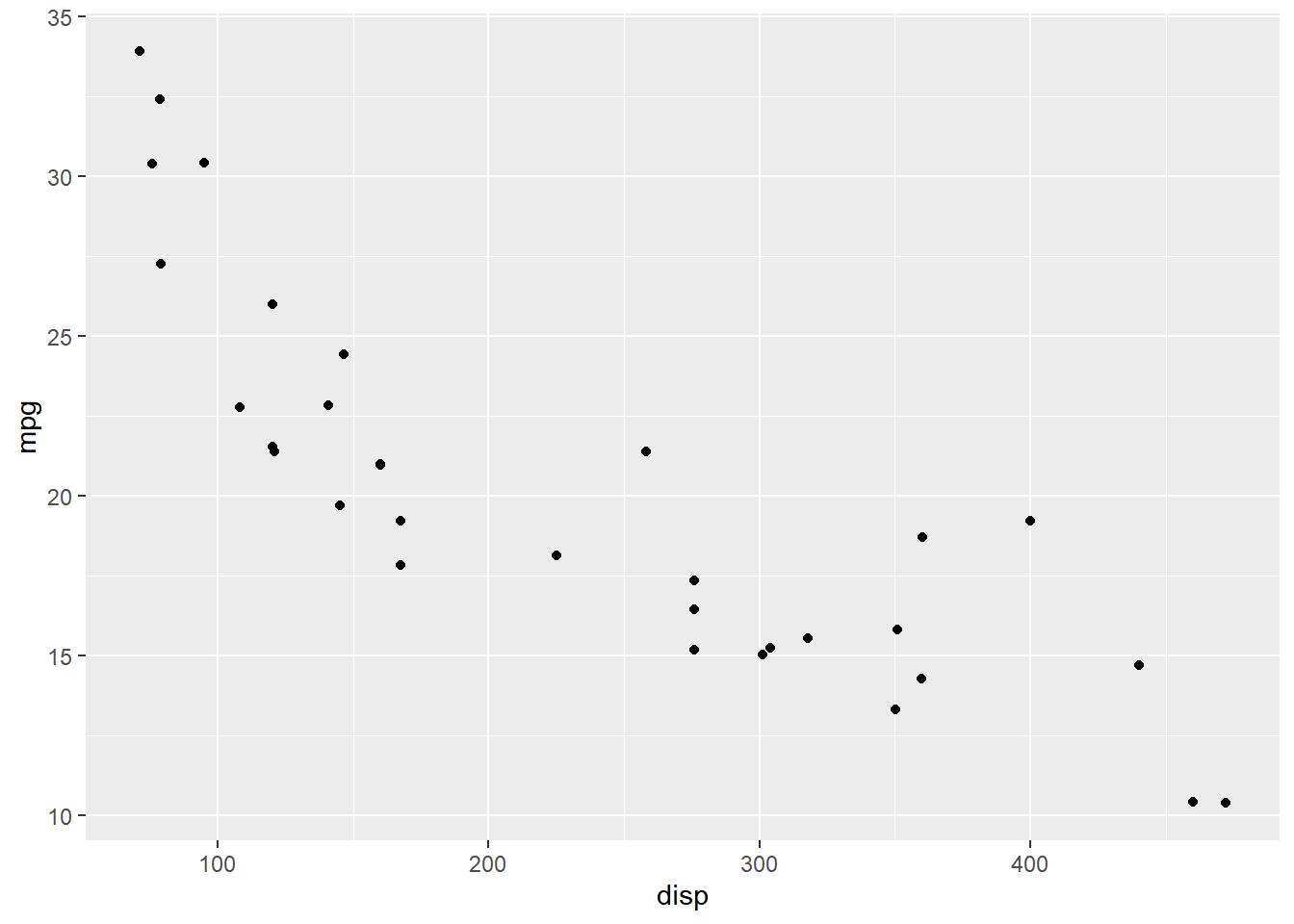Another way to avoid over plotting is to use `geom_jitter()`.

``````ggplot(mtcars) +
geom_jitter(aes(disp, mpg))``````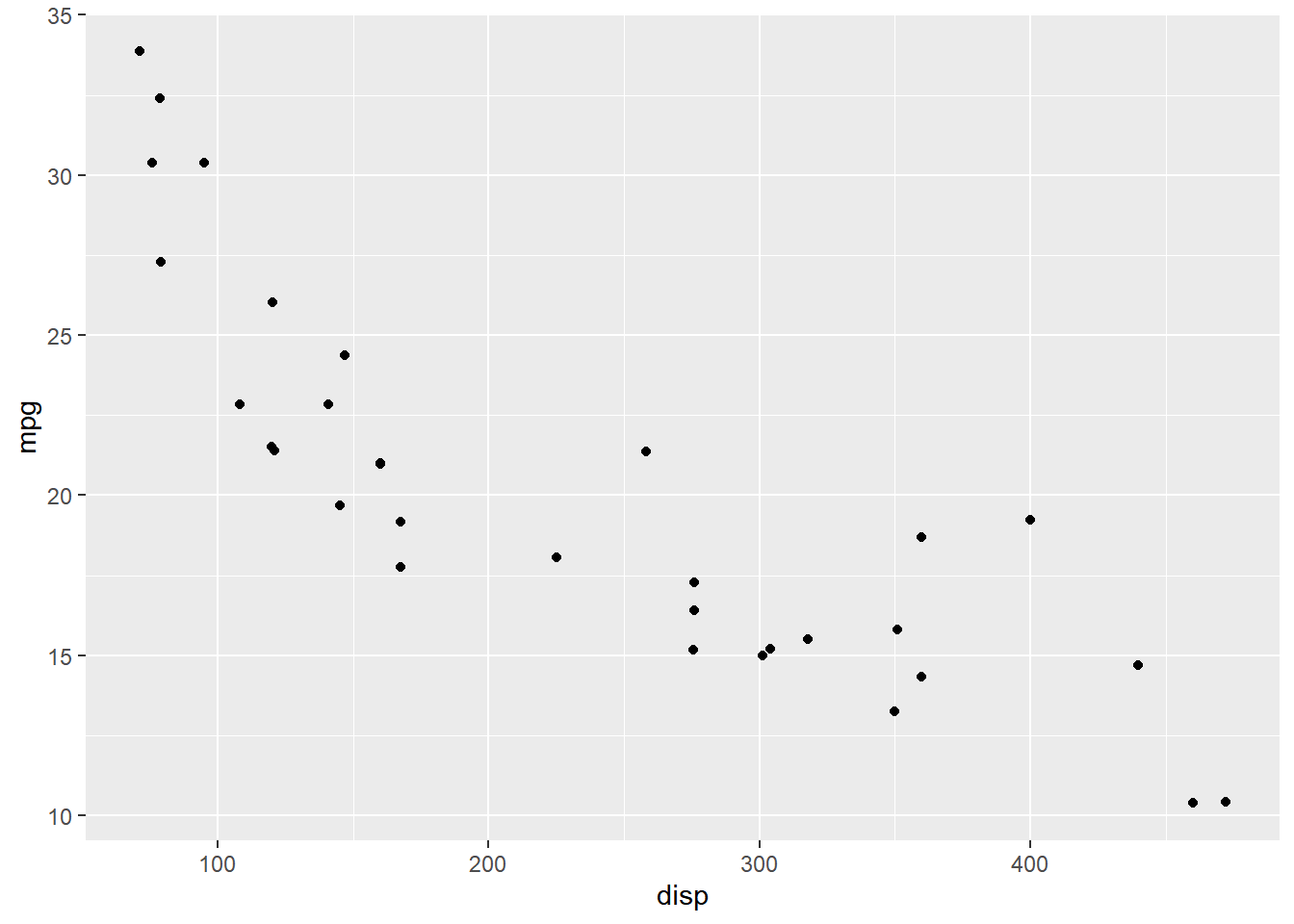#### Aesthetics

Now let us modify the appearance of the points. There are two ways:

• specify values
• map them to variables using `aes()`

#### Specify Values

##### Color

To modify the color of the points, you can use the `color` argument and supply it a valid color name. In the below example, we change the color of the points to `'blue'`. Keep in mind that the `color` argument should be outside `aes()`.

``````ggplot(mtcars) +
geom_point(aes(disp, mpg), color = 'blue', position = 'jitter')``````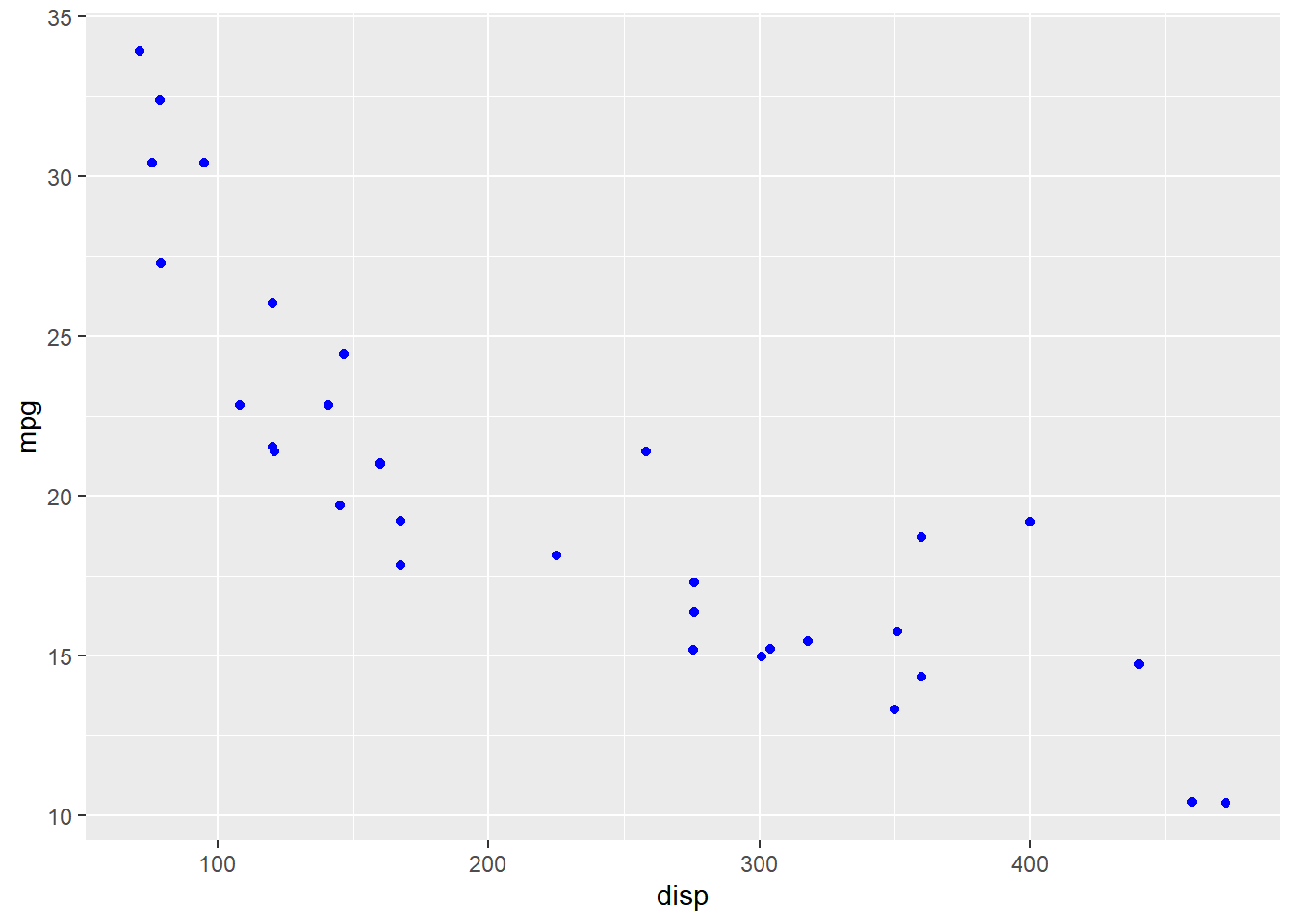##### Alpha

The transparency of the color can be modified using the `alpha` argument. It takes values between `0` and `1`.

``````ggplot(mtcars) +
geom_point(aes(disp, mpg), color = 'blue', alpha = 0.4, position = 'jitter')``````##### Shape

The shape of the points can be modified using the `shape` argument. It takes values between `0` and `25`.

``````ggplot(mtcars) +
geom_point(aes(disp, mpg), shape = 3, position = 'jitter')``````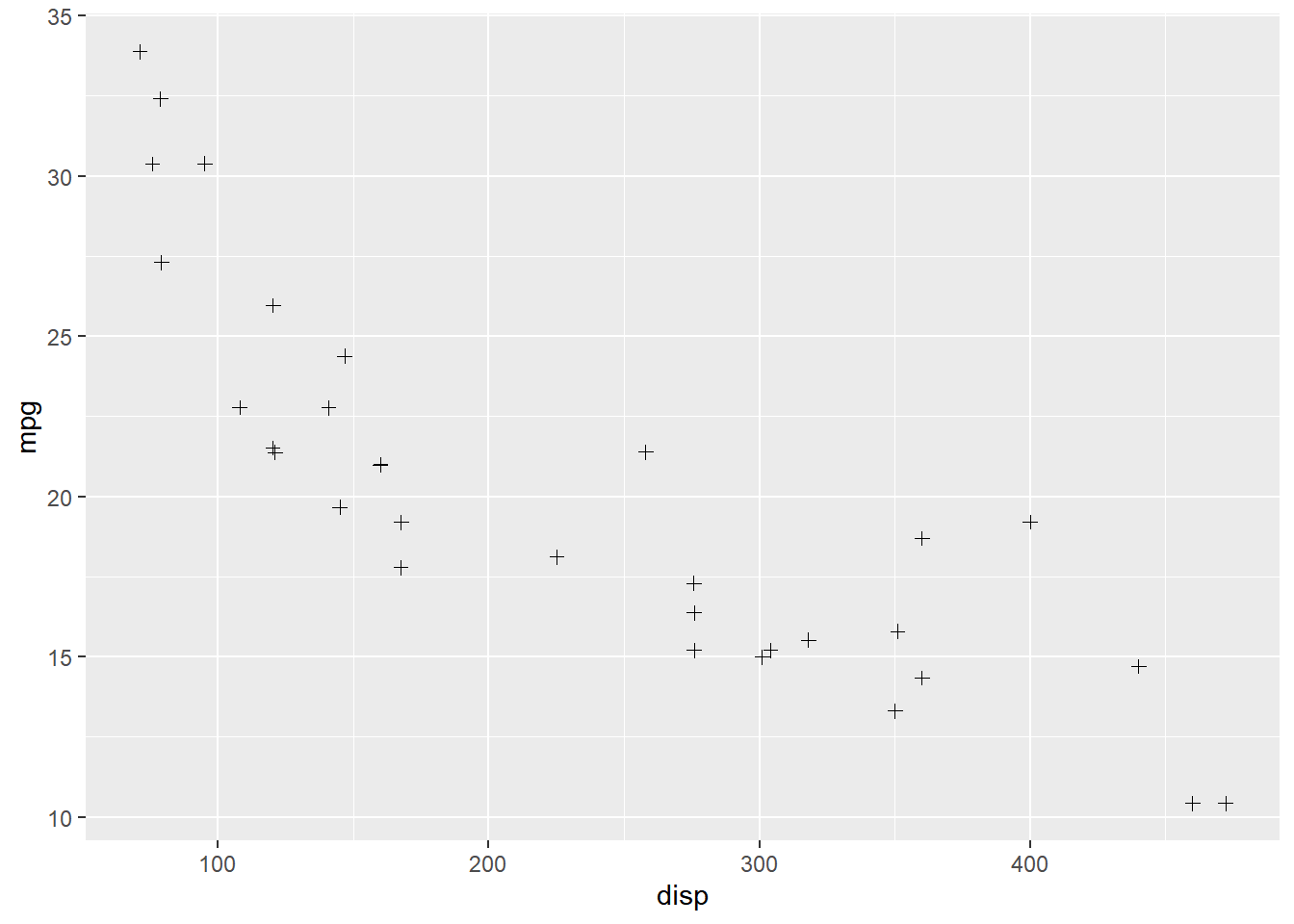##### Size

The size of the points can be modified using the `size` argument. It can take any value greater than `0`.

``````ggplot(mtcars) +
geom_point(aes(disp, mpg), size = 3, position = 'jitter')``````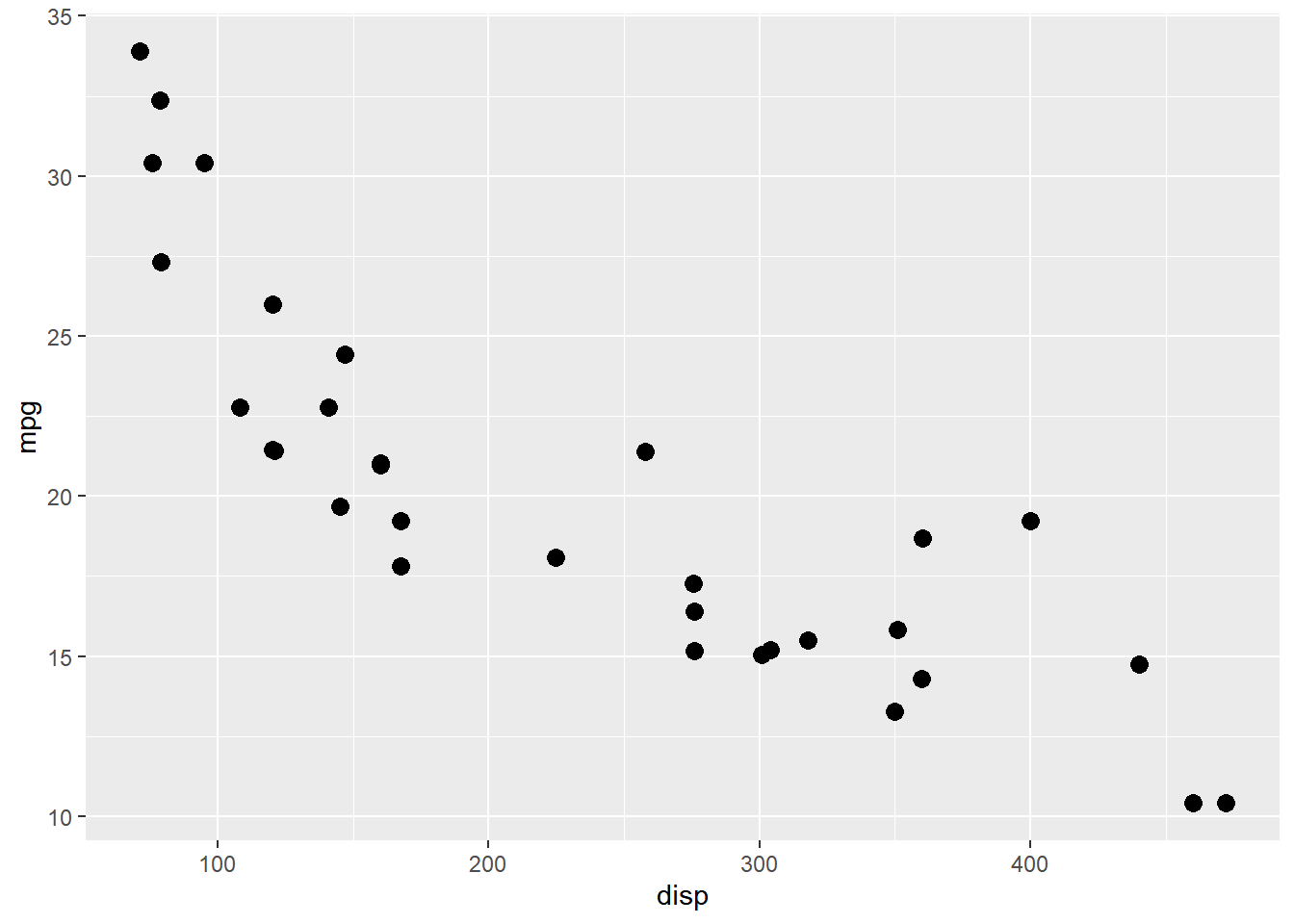#### Map Variables

So far, we have specified values for color, shape, size etc. Now, let us map them to variables using `aes()`.

##### Color

You can modify the color of the points by mapping them to a variable using `aes()`. It allows you to examine the relationship between two continuous variables at different levels of a categorical variable.

``````ggplot(mtcars) +
geom_point(aes(disp, mpg, color = factor(cyl)),
position = 'jitter')``````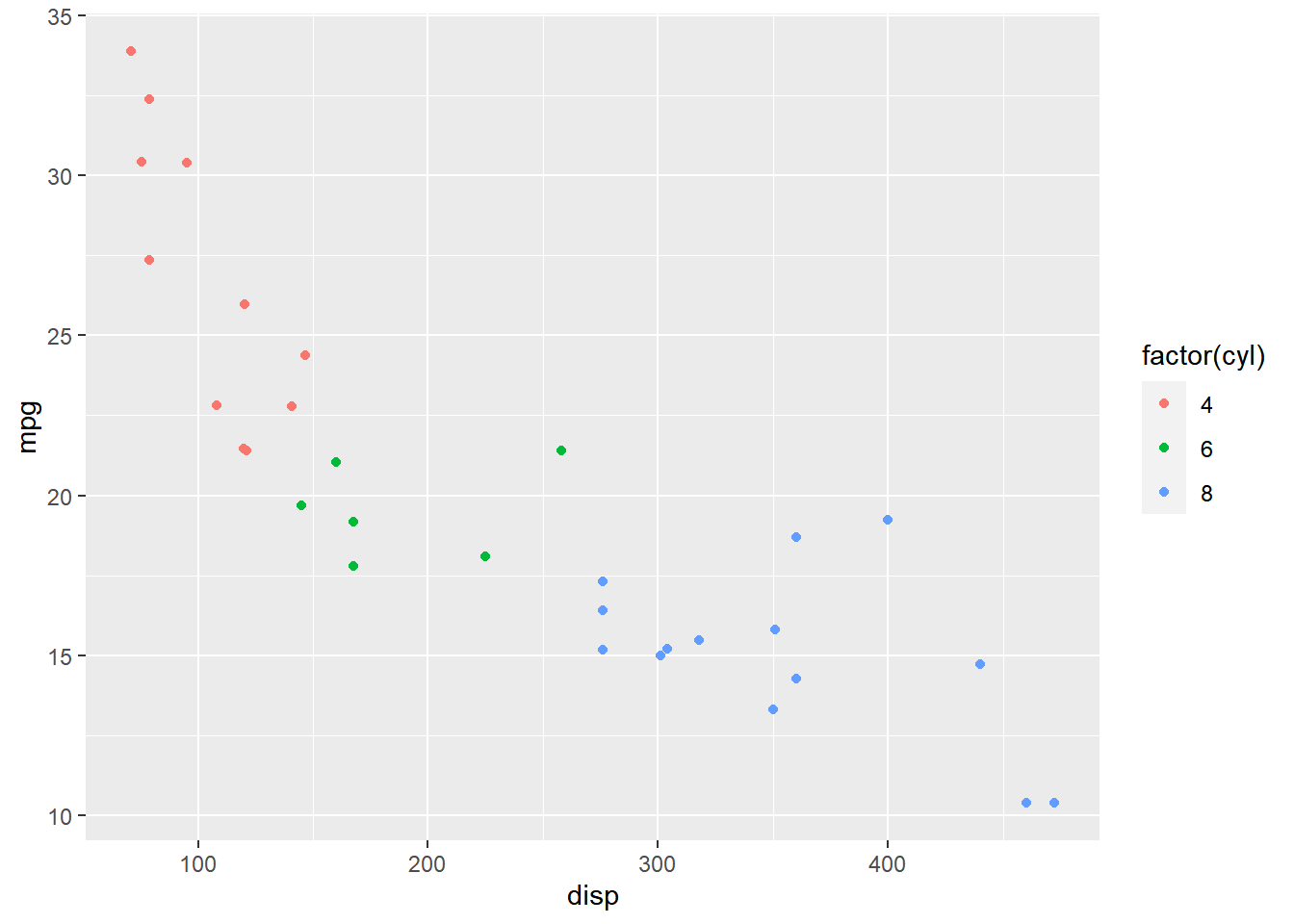The color can be mapped to a conitnuous variable as well and in this case you will be able to examine the relationship betweem two continuous variable for a range of value of a third variable.

``````ggplot(mtcars) +
geom_point(aes(disp, mpg, color = hp),
position = 'jitter')``````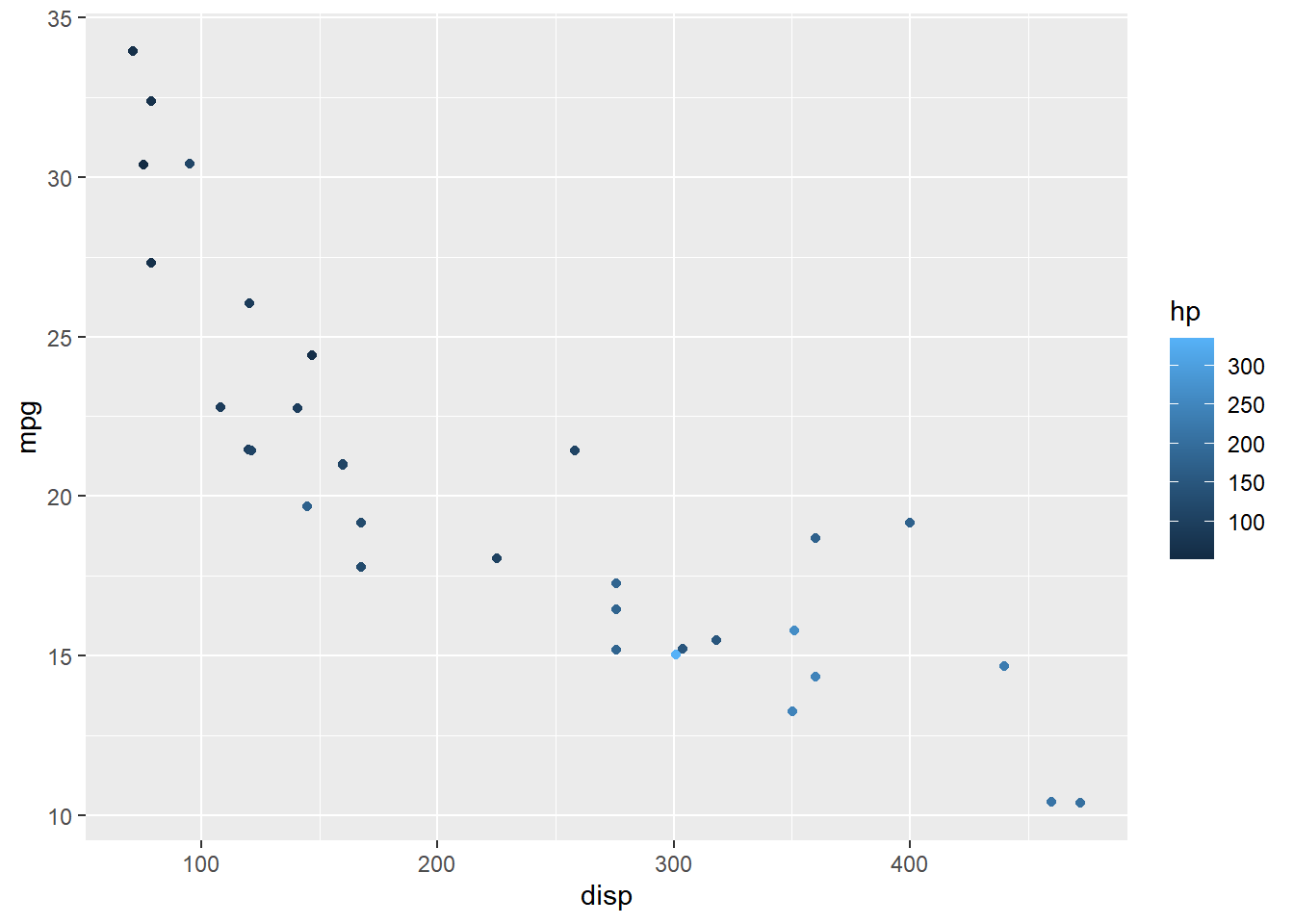##### Shape

Shape can be mapped to categorical variables. In the below example, we use `factor()` to convert `cyl` to categorical data before mapping shape to it. ggplot2 will throw an error if you map shape to a continuous variable.

``````ggplot(mtcars) +
geom_point(aes(disp, mpg, shape = factor(cyl)), position = 'jitter')``````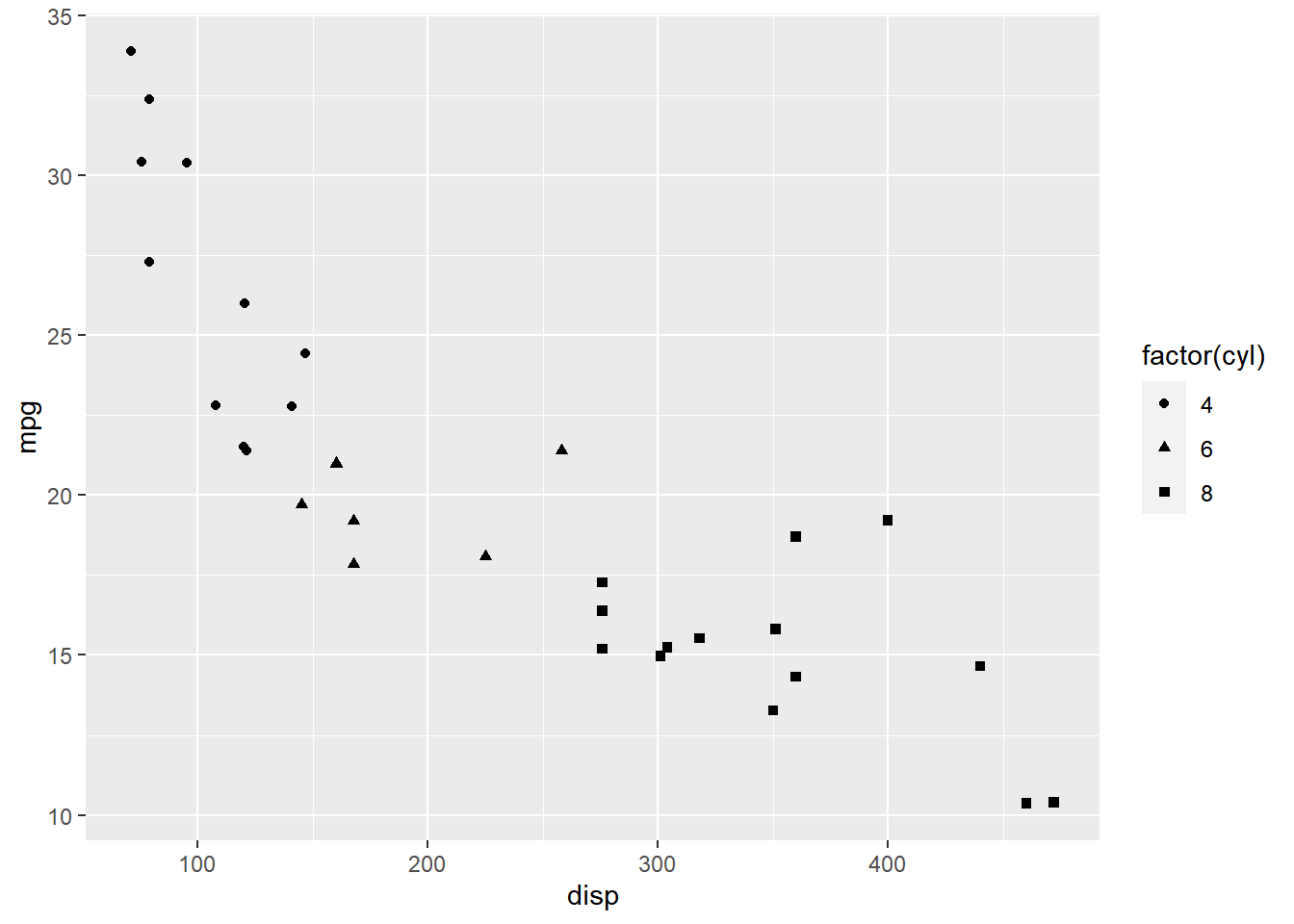##### Size

Size must be always mapped to continuous variables. In the below example, we have mapped size to `hp` variable.

``````ggplot(mtcars) +
geom_point(aes(disp, mpg, size = hp), color = 'blue', position = 'jitter')``````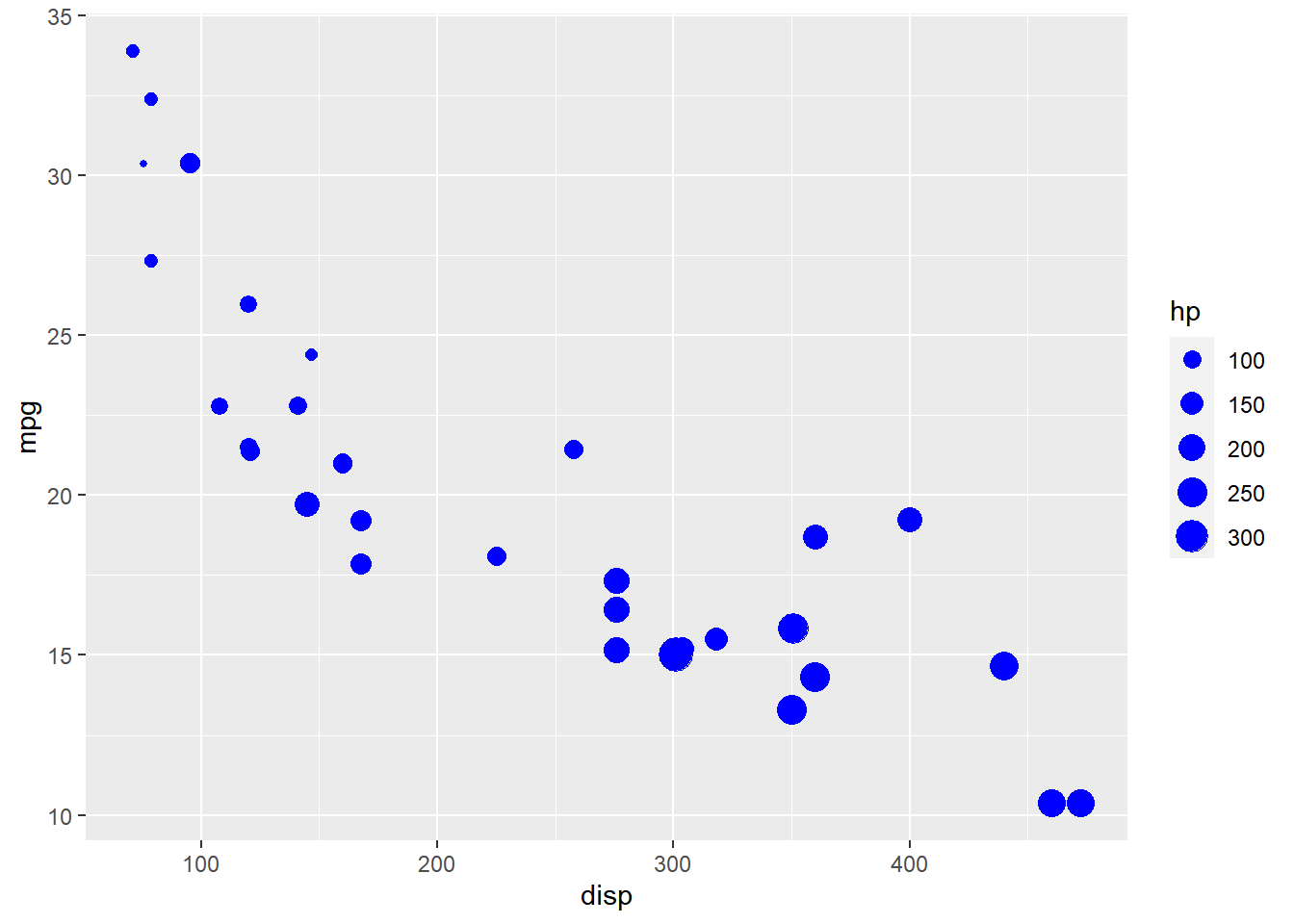If you map size to categorical data as shown in the below example, ggplot2 will throw a warning.

``````ggplot(mtcars) +
geom_point(aes(disp, mpg, size = factor(cyl)), color = 'blue', position = 'jitter')``````
``## Warning: Using size for a discrete variable is not advised.``#### Regression Line

`geom_smooth()` allows us to fit a regression line to the plot. By default it will use least squares method to fit the line but you can also use the loess method. In the below example, we fit a regression line using the least squares technique by supplying the value `'lm'` to the `method` argument.

``````ggplot(mtcars, aes(disp, mpg)) +
geom_point(position = 'jitter') +
geom_smooth(method = 'lm', se = FALSE)``````
``## `geom_smooth()` using formula 'y ~ x'``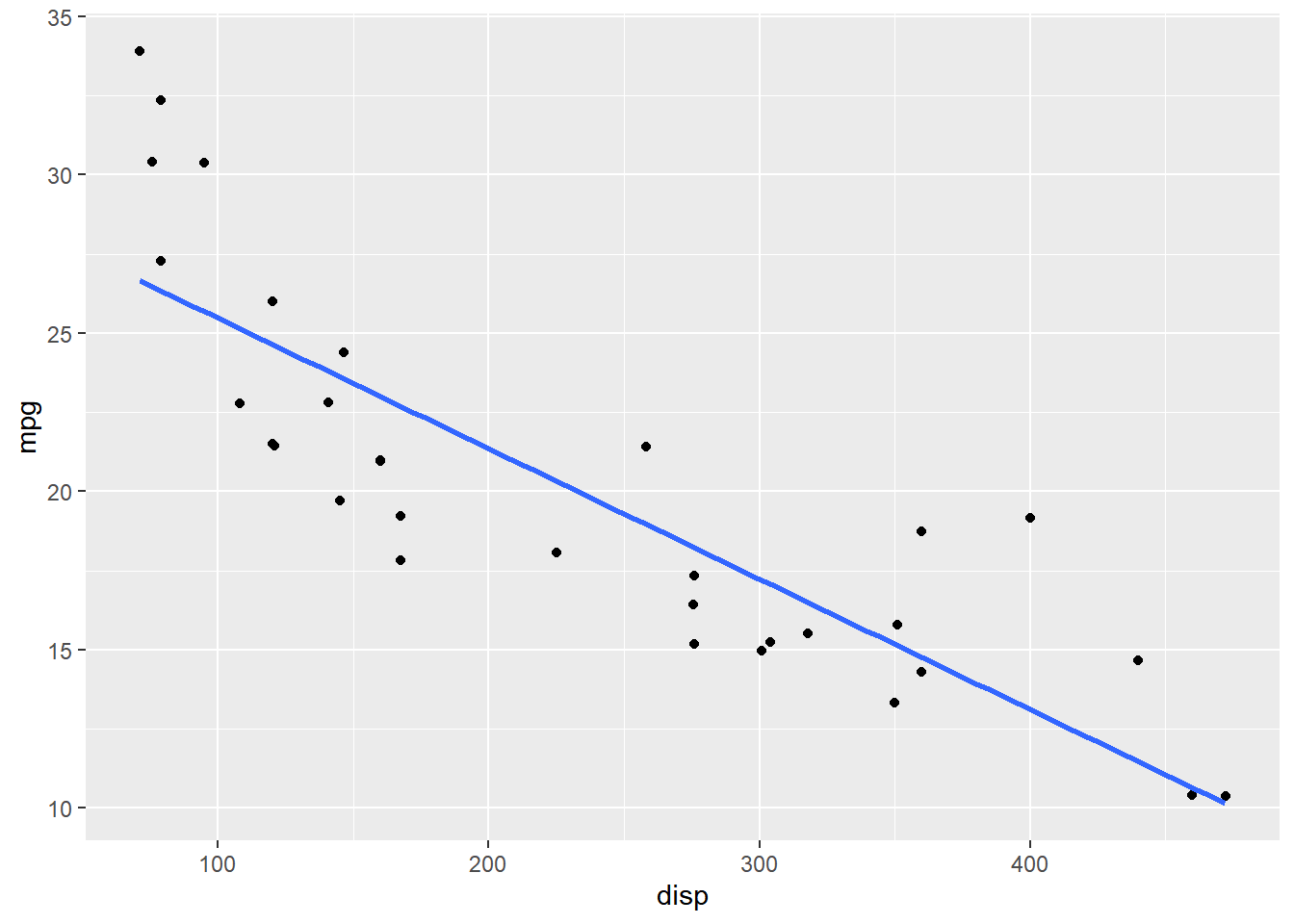#### Intercept & Slope

If you know the intercept and the slope of the line, you can use `geom_abline()`. Let us regress `mpg` over `disp` and then use the result to add the line.

###### Regression
``lm(mpg ~ disp, data = mtcars)``
``````##
## Call:
## lm(formula = mpg ~ disp, data = mtcars)
##
## Coefficients:
## (Intercept)         disp
##    29.59985     -0.04122``````
``````ggplot(mtcars, aes(disp, mpg)) +
geom_point(position = 'jitter') +
geom_abline(slope = -0.04122, intercept = 29.59985)``````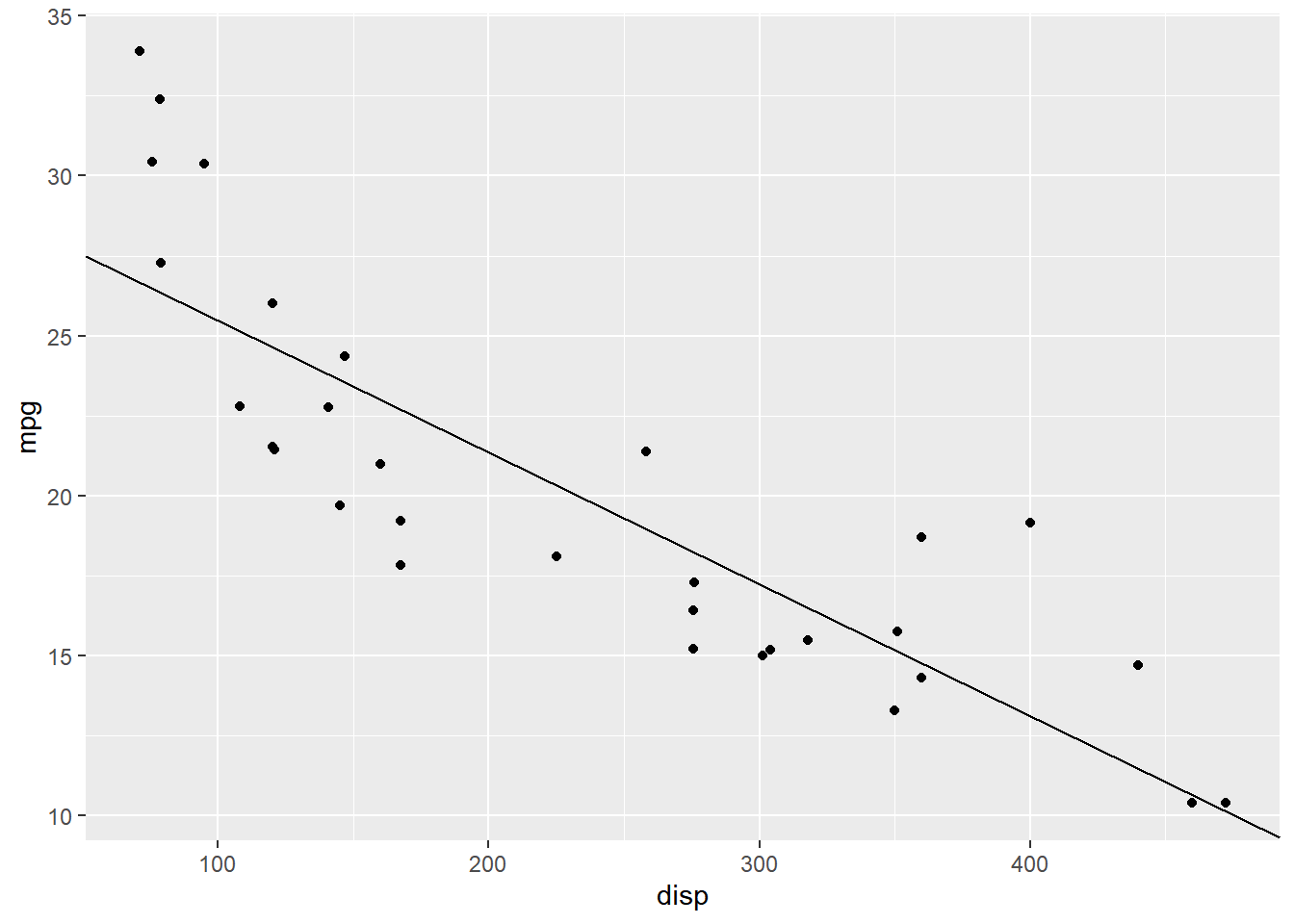The `se` argument will add a confidence interval around the regression line, if set to `TRUE`.

##### Conf. Interval
``````ggplot(mtcars, aes(disp, mpg)) +
geom_point(position = 'jitter') +
geom_smooth(method = 'lm', se = TRUE)``````
``## `geom_smooth()` using formula 'y ~ x'``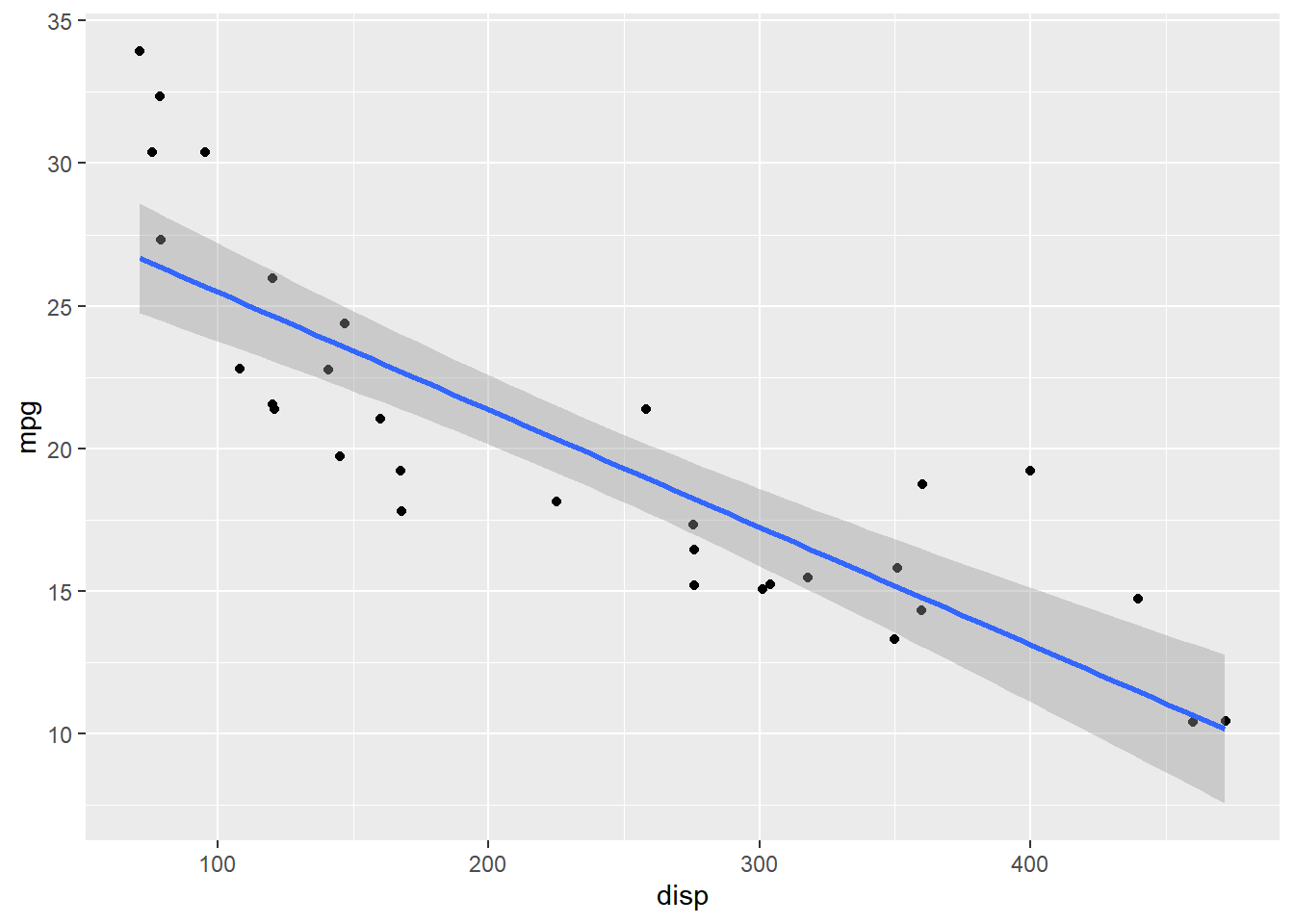##### Loess Method

In the below example, we use the loess method instead of the default least squares method to fit the regression line.

``````ggplot(mtcars, aes(disp, mpg)) +
geom_point(position = 'jitter') +
geom_smooth(method = 'loess', se = FALSE)``````
``## `geom_smooth()` using formula 'y ~ x'``### Summary

In this post, we learnt to:

• build scatter plots
• map aesthetics to variables
• fit regression line

### Up Next..

In the next post, we will learn to build line charts.